Two looms

On two different looms with different performance can be make required quantity of textile in 6 hours when both work together. On the first machine this quantity of the textile can make in 10 hours. How long should take make same quantity of textile on a second machine?

Result

b =  15 h

Solution:

b/6-b/10=1

2b = 30

b = 15

Calculated by our simple equation calculator.

Leave us a comment of example and its solution (i.e. if it is still somewhat unclear...):Be the first to comment!To solve this example are needed these knowledge from mathematics:

Do you have a linear equation or system of equations and looking for its solution? Or do you have quadratic equation?

Next similar examples:

1. Working together on projectTwo employees working on the project. First make it alone for 20 days the second for 24 days. How many days will take to complete project if 5 days they work together then first will take two days holiday and after returning they completes a project toget
2. Four painters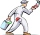The company sent four painters to paint the school. They should be done in 12 days. After three days, one painter got ill. How long will the remaining painters paint the school?
3. Hectoliters of waterThe pool has a total of 126 hectoliters of water. The first pump draws 2.1 liters of water per second. A second pump pumps 3.5 liters of water per second. How long will it take both pumps to drain four-fifths of the water at the same time?
4. Digging companies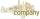Company A would dig a pit for 12 days, company B for 15 days, company C for 20 days and the company D for 24 days. Work began together companies C and D, but after three days joined them other two companies. How long took to dig a pit?
5. Six workersSix workers planned to repair the roof in 5 days. After two days, two workers get sick. How long will the remaining workers complete the roof at the same rate of work?
6. Apprentice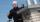Worker and apprentice execute the work for 6 hours. Worker performs himself in 10 hours. How long do the work of an apprentice?
7. Six yearsIn six years Jan will be twice as old as he was six years ago. How old is he?
8. Our houseOur house would paint four painters in 14 days. How long could it paint five painters? How long will it take if two other painters will come to help after 5 days?
9. EquationSolve the equation: 1/2-2/8 = 1/10; Write the result as a decimal number.
10. Painters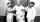One painter could paint a school for 7 days. The second one for 6 days. It was necessary to paint the school in two days. Next painter was invited and all worked together. How long would this painter painted the school itself?
11. Collection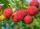John has collected half a bucket of raspberries in 45 minutes, Eva collects the whole bucket in an hour. How many minutes it would take to fill a bucket if the two children work together?
12. Pumps A and BPump A fill the tank for 12 minutes, pump B for 24 minutes. How long will take to fill the tank if he is only three minutes works A and then both pumps A and B?
13. Fifth of the numberThe fifth of the number is by 24 less than that number. What is the number?
14. Two tributaries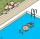Two tributaries of the pool fill it in 10 hours. One of the tributaries would fill 15 hours. How long would fill the first tributary?
15. Simple equationSolve for x: 3(x + 2) = x - 18
16. Unknown numberIdentify unknown number which 1/5 is 40 greater than one tenth of that number.
17. Three peopleThree people start doing a work at a same time. The first worked only 2 hours. The second ended 3 hours before the end. On an individual basis, it would take the first time to do the work 10 hours, second 12 hours and 15 hours third. How many hours did it.